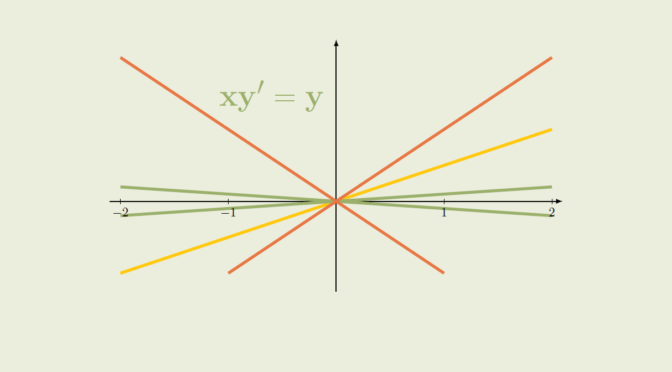A linear differential equation with no solution to an initial value problem

Consider a first order linear differential equation $y^\prime(x) = A(x)y(x) + B(x)$ where $$A, B$$ are real continuous functions defined on a non-empty real interval $$I$$. According to Picard-Lindelöf theorem, the initial value problem $\begin{cases} y^\prime(x) = A(x)y(x) + B(x)\\ y(x_0) = y_0, \ x_0 \in I \end{cases}$ has a unique solution defined on $$I$$.

However, a linear differential equation $c(x)y^\prime(x) = A(x)y(x) + B(x)$ where $$A, B, c$$ are real continuous functions might not have a solution to an initial value problem. Let’s have a look at the equation $x y^\prime(x) = y(x) \tag{E}\label{eq:IVP}$ for $$x \in \mathbb R$$. The equation is linear.

For $$x \in (-\infty,0)$$ a solution to \eqref{eq:IVP} is a solution of the explicit differential linear equation $y^\prime(x) = \frac{y(x)}x$ hence can be written $$y(x) = \lambda_-x$$ with $$\lambda_- \in \mathbb R$$. Similarly, a solution to \eqref{eq:IVP} on the interval $$(0,\infty)$$ is of the form $$y(x) = \lambda_+ x$$ with $$\lambda_+ \in \mathbb R$$.

A global solution to \eqref{eq:IVP}, i.e. defined on the whole real line is differentiable at $$0$$ hence the equation $\lambda_- = y_-^\prime(0)=y_+^\prime(0) = \lambda_+$ which means that $$y(x) = \lambda x$$ where $$\lambda=\lambda_-=\lambda_+$$.

In particular all solutions defined on $$\mathbb R$$ are such that $$y(0)=0$$. Therefore the initial value problem $\begin{cases} x y^\prime(x) = y(x)\\ y(0)=1 \end{cases}$ has no solution.